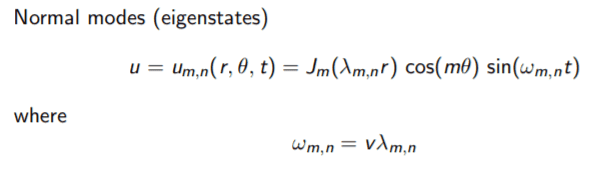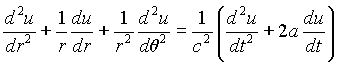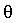# Calculating decay rates for modes of a circular membrane

• A
• mikejm
In summary, the conversation is about solving for the theoretical relative decay rates of (m,n) modes of an ideal circular membrane when excited by an impulse or deformation. The goal is to find the decay rates in dB/s and an equation that takes into account the excitation position and damping coefficient. The suggested method involves using plate theory to generate a PDE and solving for eigenmodes. However, the website provided for the damped wave equation does not account for frequency/mode dependent per-partial damping and may not accurately represent the decay of the membrane. The individual is looking for a better way to calculate the decay rates and improve the damped wave equation.f

#### mikejm

I am trying to solve for the theoretical relative decay rates of the various (m,n) modes of an ideal circular membrane, if that membrane is excited momentarily by an impulse or deformation.

I would ideally like the decays of the (m,n) modes in dB/s.

Imagine a simple isolated drum head being struck by a stick. The membrane should be considered fixed with even tension around its perimeter. The excitation impulse/deformation should be at its center or x*radius from its center.

Someone on another site said of this problem:

If the air damps it linearly enough, you can probably solve it analytically. Use plate theory to generate a PDE, then work out all the eigenmodes. The decay rate will be determined by the real components of the eigenvalues, and can be converted into dBs-1 using a few logs.

The wave equation for modes of an ideal circular membrane is given by:The full wave equations are described/explained further in these documents:

http://www.math.ubc.ca/~nagata/sci1/drum.pdf
https://courses.physics.illinois.ed...P406POM_Lecture_Notes/P406POM_Lect4_Part2.pdf
http://ramanujan.math.trinity.edu/rdaileda/teach/s12/m3357/lectures/lecture_3_29.pdf

I can use the Bessel zeros to calculate the frequencies of the various (m,n) modes and have done so already. However, I am unsure how to get the decay rates for these modes as he describes.

Does the method he suggests make sense? If so, can anyone elaborate further on how I would go about doing this? Or is there a better way?

Ideally I'd like an equation I can put in (m,n) for, plus perhaps an arbitrary constant damping coefficient, and get the decay of that mode in dB/s. If the decay rate of any mode might vary depending on the point of excitation, some way to specify for excitation position might be useful.

Thanks for any help!

#### Attachments

I was able to find a website summarizing a damped wave equation for a circular membrane here:where u is the amplitude of vibration of the membrane, r andare polar coordinates of membrane, a is the damping factor, c is the speed of a wave on the membrane.

http://www.math.ust.hk/~machas/drum/

However, the membrane simulation sounds terrible (audio clips at the end of that site) because they aren't employing the proper frequency/mode dependent per-partial damping that occurs in nature.

As far as I can tell, this is just damping all the modes equally over time, which is useless.

Am I understanding this correctly, and if so, is there any obvious way to improve this and get a more representative damped wave equation?